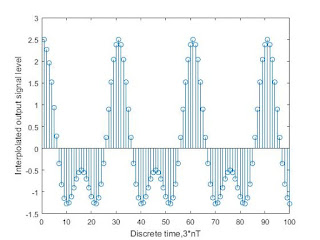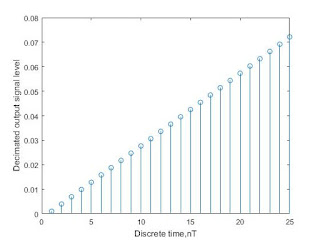Impact-Site-Verification: dbe48ff9-4514-40fe-8cc0-70131430799e

## Search This Blog

Consider a C.T signal

x(t)=a*cos(2*pi*f1*t)+b*cos(2*pi*f1*t)

if f1=100Hz
f2=200hz
a/b=1.5

You will get answers of following questions:
1. Generation of DT signal
2. Interpolate the signal by factor I=3
3. Decimate this interpolated signal by factor D=3
4. Plot all the signal

### MATLAB PROGRAM:

clear all;

close all;

fs=1000; %Sampling frequency

a=1.5;  %relative amplitudes

b=1;

f1=100; %Values of f1 and f2

f2=200;

t=0:1/fs:1; %time vector

x=a*cos(2*pi*f1*t)+b*cos(2*pi*f2*t);    %generation of x(t);

y=interp(x,3); %interpolate signal by 3

stem(x(1:25));  %plot original signal

xlabel('Discrete time,nT')

ylabel('Input signal level');

figure

stem(y(1:100));         %plot interpolated signal

xlabel('Discrete time,3*nT')

ylabel('Interplolated output signal level');

figure;

y1=decimate(t,3);

stem(y1(1:25));  %plot decimated signal

xlabel('Discrete time,nT')

ylabel('Decimated output signal level');

### OUTPUT:Original Signalinterpolated signaldecimated signal